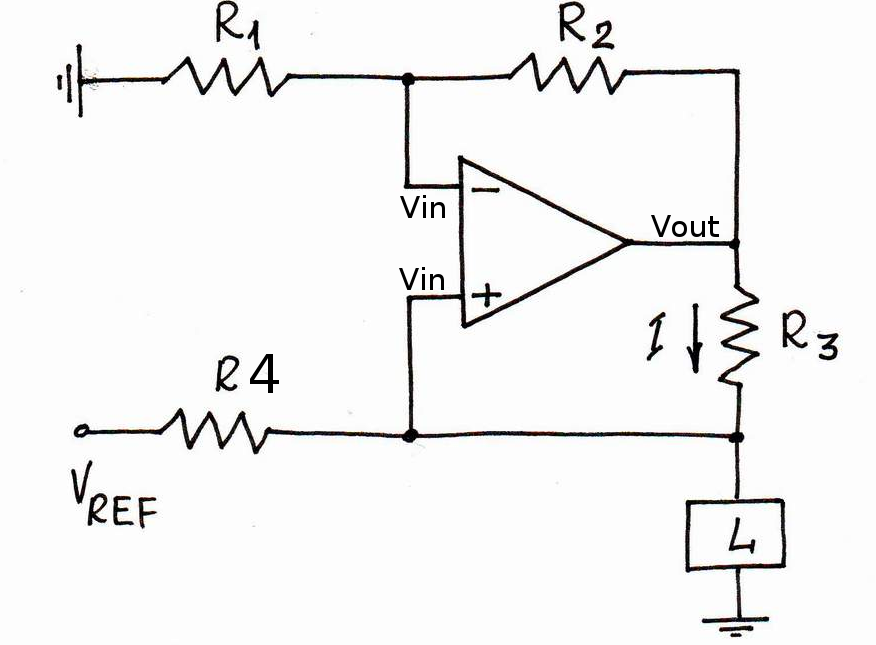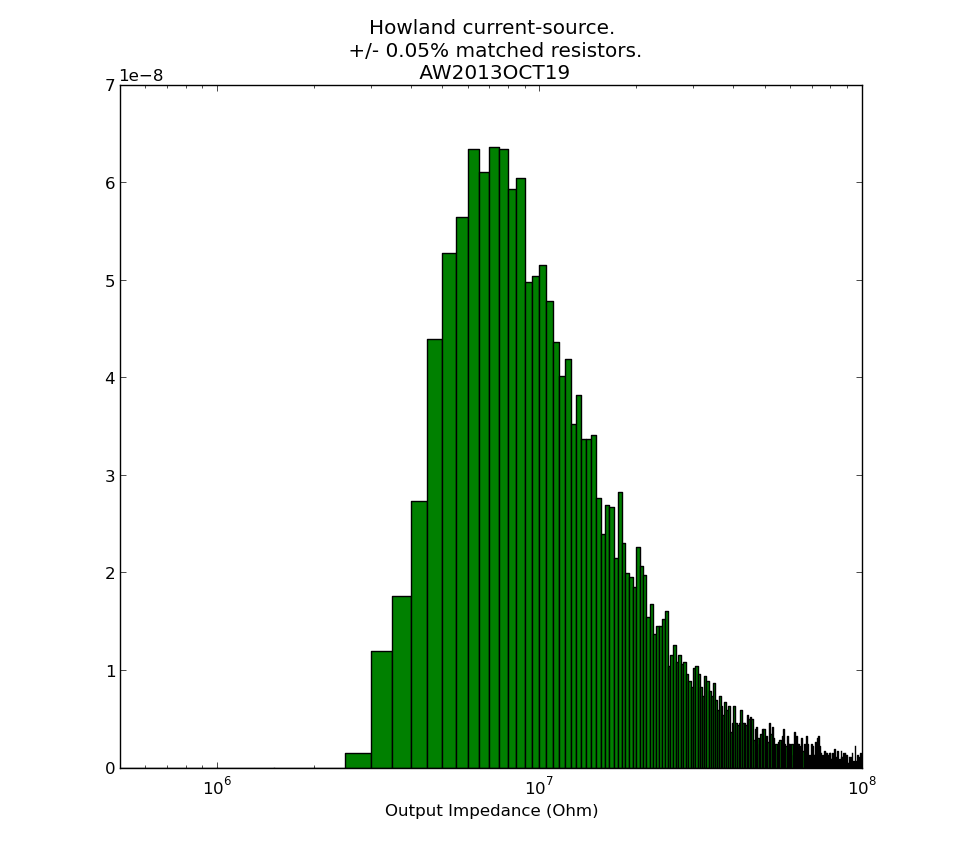## Howland constant current source

For pushing a constant 500 uA current through Pt100 temperature sensors I am using a Howland constant current source.I'm using an OP2177 op-amp. The OPA2188 would have been better, but the delivery time was too long. The four resistors come in a single 4x10k array package, and are matched to within +/-0.05 % (ACASA1002E1002P100). The reference voltage comes either from an external source, or an on-board 5V ADR425.

We did a test of how good the current source is, and came up with this data:The slope corresponds to an output impedance of 3.6 MOhms. Note that when we change the load resistance from 0 Ohms to 600 Ohms the load current changes by 83 nA, or less than 0.02% of full-scale (500uA). The 6.5-digit multimeter in the electronics lab was mostly oblivious to this change, so we used a 3458A instead 🙂

I then wanted to calculate what contribution the tolerance of the resistors R1-R4 make to the output impedance. Assuming an ideal op-amp (infinite gain, so V+ and V- at same voltage), the op-amp output voltage can be seen to be (left as an exercise for the reader):

Now we can make a monte-carlo simulation by picking values for R1-R4 with a nominal 10k resistance and an added +/-0.05% tolerance. I did this by adding a normal-distributed resistance with zero mean and 5 Ohm std-deviation.

I then repeated the output impedance calculation many times, and plotted this output impedance histogram:It seems our measured 3.6 MOhms is slightly low, and from the resistors alone something closer to 10 MOhm should be expected. Non-ideal behaviour of the op-amp, which I haven't considered here, may also contribute. Python-script for producing the histogram: howland_calc.py

See also: AN-1515 A Comprehensive Study of the Howland Current Pump (up to 1 MOhm output-impedance with 1% resistors is mentioned)

This current-source is part of the Pt100 frontend.